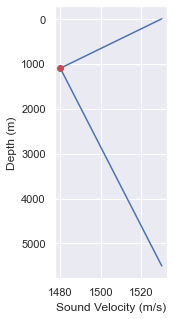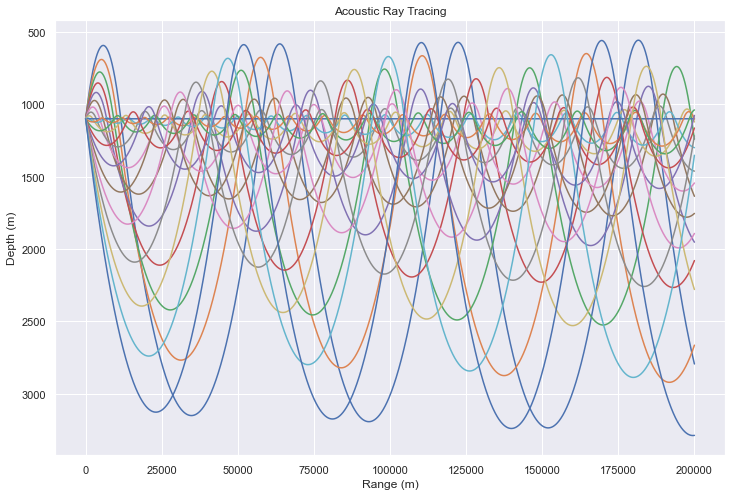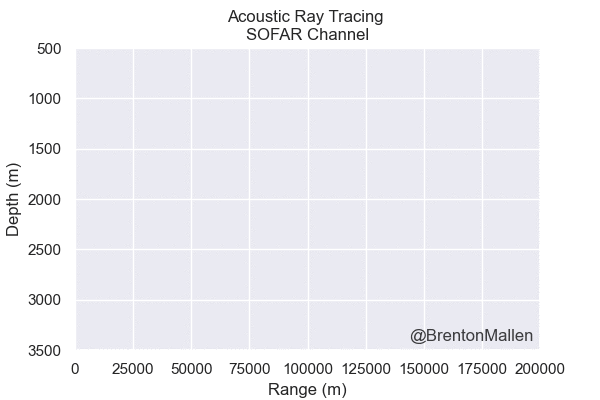rat_trace_blog

# Underwater Acoustic Ray Tracing in the SOFAR Channel using Python¶

In grad school, one homework assignment we had was to perform an acoustic ray trace of the SOFAR channel. This was done in MATLAB and I wanted to revisit it for a couple of reasons: MATLAB isn't really accessible (there is octave, but I feel python is much more approachable) and I miss working with acoustics every now and then. So with that in mind, I thought I could use my python programming powers along with my yearning to do some acoustic stuff to work through this homework assignment again.

What is ray tracing?

Ray tracing) is simply that, figuring out (tracing) that path taken by a source to its destination. It can be applied to both sound and light. In the world of acoustics, it can be used to model sound performance in a concert hall or even, as will be shown here, the path sonar would take through a body of water. It is also used in 3D rendering - tracing out the paths of light from a source to determine how light interacts with objects in the scene and how the resulting image should appear.

Note: This is by no means a treatise on the subject as there's a lot behind getting to this point but I will attempt to provide a concise look into the main mechanisms going on here. There are also links to supplemental material scattered throughout should you wish to explore further.

Resource:

A really good resource on the work explored here can be found in this chapter of a book entitle Modeling and Measurement Methods for Acoustic Waves and for Acoustic Microdevices by Jens M. Hovem. There are also a number of books out there on the subject including Principles of Underwater by Robert J. Urick.

In :
import numpy as np
import matplotlib.pyplot as plt
%matplotlib inline
import seaborn as sns
sns.set()
from tqdm import tqdm

from IPython.display import HTML, display, Image
from matplotlib import animation

# load custom css to center all images in output html

Out:

### Speed of Sound in the Ocean¶

An example of the sound velocity profile within a water column can be seen in Figure 1. The speed of sound underwater is a function of temperature, salinity and depth. Typically, temperature will decrease and salinity will increase with depth. The water's temperature and salinity tend to go through a period of drastic change (closer to the surface with environmental factors have greater impact). These layers of high gradient are called the thermocline and halocline, respectively and gradient will decrease with depth after these layers.

The speed of sound is typically measured empirically by performing by using an intstrament called a CTD) to gather salinity (C), temperature (T) and depth (D) data. From this data, a choice of arguably byzantine looking equations can be used to determing sound speed.Figure 1: Example Sound Velocity Profile

So why do we care about the sound speed profile? Well, it can have a big impact on the path sound waves will travel, especially the change in sound speed between layers. (Layers, in this context, are discrete slices in the water column parallel to surface.) Understanding the path sound waves take in the water can help with applications such as sonar and acoustic communication.

Side note: If you'd like to explore sound velocity profile data, you can download some data from NOAA! (bonus if you're a R programmer)

### SOFAR Channel¶

One really neat thing about the composition of a sound velocity profile like the one shown in Figure 1 is that it allows for the creation of a layer known as the SOFAR (Sound Fixing and Ranging) channel. This channel, which we'll model below, is a layer that allows for very long propagation of sound waves due to a phenomena known as total internal reflection as sound waves experience a 'steering' described by Snell's Law.

## Modeling the SOFAR Channel¶

First a few parameters will be set to establish the conditions in this model, specifically the minimum and maximum sound speeds along with depth of the water column.

In :
# SPEED_OF_SOUND_SURFACE = 1480  # meters / second
SOUND_SPEED_MIN = 1480
SOUND_SPEED_MAX = 1530

DEPTH_MIN = 0  # Ocean Surface
DEPTH_MAX = 5500  # meters
DEPTH_MIN_SPEED = 1100 # depth where minimum speed of sound is observed
DEPTH_RANGE = np.arange(DEPTH_MIN, DEPTH_MAX + 1)


In order to develop a ray tracing model of the SOFAR channel, an assumption is made that the sound velocity profile is linear in two sections. The first section, nearer the surface, has a decreasing sound speed while the second section, continuing into the depth, has an increasing one. For each of these sections, we'll determine their sound velocity gradient. This value will be constant due to our linear assumption.

In :
SOUND_GRADIENT_SHALLOW = (SOUND_SPEED_MIN - SOUND_SPEED_MAX) / (DEPTH_MIN_SPEED - DEPTH_MIN)
SOUND_GRADIENT_DEEP = (SOUND_SPEED_MAX - SOUND_SPEED_MIN) / (DEPTH_MAX - DEPTH_MIN_SPEED)

# sound velocity gradient up to 1100 meters deep
(1, np.argwhere(DEPTH_RANGE == DEPTH_MIN_SPEED)),
)
# sound velocity gradient beyond 1100 meters
(1, np.argmax(DEPTH_RANGE) - np.argwhere(DEPTH_RANGE == DEPTH_MIN_SPEED)),
)

# construct the sound velocity profile
sound_velocity_profile = SOUND_SPEED_MIN + \
(DEPTH_RANGE[:-1] - DEPTH_MIN_SPEED) * \

# plot the sound velocity profile
fig = plt.figure(figsize=(2,5))
plt.plot(sound_velocity_profile, DEPTH_RANGE[:-1])
plt.plot([SOUND_SPEED_MIN],[DEPTH_MIN_SPEED], 'ro')
plt.gca().invert_yaxis()
plt.ylabel('Depth (m)')
plt.xlabel('Sound Velocity (m/s)')
plt.show()

HTML("""<center>
Figure 2: Theoretical Sound Velocity Profile
<br>
(The red dot is the acoustic source.)
</center>""")Out:
Figure 2: Theoretical Sound Velocity Profile
(The red dot is the acoustic source.)

Now that we have our sound velocity profile, to illustrate the SOFAR channel, an acoustic source is placed at the point of lowest sound speed. Figure 2 illustrates a red point where the source is located. The sound transmission from the source is discretized to a small set of angles to reduce computation complexity. We discretize the transmission angles we will be simulating from -10 to 10 degrees relative to the horizontal at the source depth.

In :
SOURCE_DEPTH = DEPTH_MIN_SPEED
SOURCE_SOUND_SPEED = SOUND_SPEED_MIN
angle_0_ind = np.argwhere(TRANSMISSION_ANGLE_RANGE == 0)  # index of the 0 degree mark


For the purposes of this simulation, we'll set the maximum distance to 200,000 meters and discretize that into steps of 20 meters.

In :
SIMULATION_STEPS = 20  # meters
SIMULATION_RANGE = np.arange(0, 200e3 + SIMULATION_STEPS, SIMULATION_STEPS)


## Tracing A Ray Path¶

Given a sound wave from the source at a transmission angle ($\phi$), we want to know the depth (z) of the wave at each point in the simulation range (r). The change in depth (dz) is a function of the radius of curvature of the sound wave (R) which is in turn influenced by the impact of the speed of sound (c) and the sound speed gradient (g) on the refraction angle ($\theta$) (due to snell's law).

\begin{align*} z_{(\phi_{i}, ~r_{j})} = z_{(\phi_{i}, ~r_{j-1})} + dz \end{align*}

\begin{align*} dz = R_{(\phi_{i}, ~r_{j-1})} ~*~(cos(\theta_{\phi_{i}, ~r_{j-1})})-cos(\theta_{(\phi_{i}, ~r_{j})})) \end{align*}

\begin{align*} c_{(\phi_{i}, ~r_{j})} = c_{source} + c_{shallow}(z_{(\phi_{i}, ~r_{j})} - z_{source}) \end{align*}\begin{align*} R_{(\phi_{i}, ~r_{j})} = -\frac{c_{(\phi_{i}, ~r_{j-1})}}{g ~* ~cos(\theta_{(\phi_{i}, ~r_{j})})} \end{align*}

## Setting Initial Conditions¶

The matrices that will contain the relevant values through the simulation need to be initialized with respect both shape and starting conditions.

In :
# Instantiate our matrices
R = np.zeros((len(TRANSMISSION_ANGLE_RANGE), len(SIMULATION_RANGE)))
z = np.zeros_like(R)
c = np.zeros_like(R)
theta = np.zeros_like(R)

# Prime the initial conditions
z[:, 0] = DEPTH_MIN_SPEED  # we put the source at the depth of min sound speed
R[:, 0] = -SOURCE_SOUND_SPEED / np.append(
)
c[:, 0] = SOUND_SPEED_MIN
theta[:, 0] = TRANSMISSION_ANGLE_RANGE


## Running the Simulation¶

This is the core of the simulation. As it iterates through each range step and transmission angle step, it calculates the appropriate parameters in order to find the depth of the sound wave at each point.

In :
for j in tqdm(range(1, len(SIMULATION_RANGE))):
for i in range(0, len(TRANSMISSION_ANGLE_RANGE)):

if (z[i, j-1] == SOURCE_DEPTH) and (theta[i, j-1] == 0):
c[i, j] = SOURCE_SOUND_SPEED
theta[i, j] = 0
dz = 0
z[i, j] = SOURCE_DEPTH

elif (z[i, j-1] < SOURCE_DEPTH) or (z[i, j-1] == SOURCE_DEPTH and theta[i, j-1] > 0):
R[i, j] = -c[i, j-1] / (SOUND_GRADIENT_SHALLOW * np.cos(theta[i ,j-1]))
theta[i, j] = np.arcsin(
SIMULATION_STEPS / R[i, j-1] + np.sin(theta[i, j-1])
)
dz = R[i, j-1] * (np.cos(theta[i, j-1]) - np.cos(theta[i, j]))
z[i, j] = z[i, j-1] + dz
c[i, j] = SOURCE_SOUND_SPEED + SOUND_GRADIENT_SHALLOW * (z[i, j] - SOURCE_DEPTH)

elif (z[i, j-1] > SOURCE_DEPTH) or (z[i, j-1] == SOURCE_DEPTH and theta[i, j-1] < 0):
R[i, j] = -c[i, j-1] / (SOUND_GRADIENT_DEEP * np.cos(theta[i ,j-1]))
theta[i, j] = np.arcsin(
SIMULATION_STEPS / R[i, j-1] + np.sin(theta[i, j-1])
)
dz = R[i, j-1] * (np.cos(theta[i, j-1]) - np.cos(theta[i, j]))
z[i, j] = z[i, j-1] + dz
c[i, j] = SOURCE_SOUND_SPEED + SOUND_GRADIENT_DEEP * (z[i, j] - SOURCE_DEPTH)


100%|██████████| 10000/10000 [00:02<00:00, 3445.24it/s]


## Plotting the Ray Trace¶

Now that we've performed all of the calculations, it's time for the fun part; visualization! Here, the the plot displays the range from the source on the x-axis and the depth on the y-axis. A nuance regarding the y-axis is that it is inverted so that depth can increase downward which is simply to help display the data in a more intuitive way.

In :
plt.figure(figsize=(12,8))
for i in range(0, len(TRANSMISSION_ANGLE_RANGE)):
plt.plot(SIMULATION_RANGE, z[i])
plt.gca().invert_yaxis()
plt.title('Acoustic Ray Tracing')
plt.xlabel('Range (m)')
plt.ylabel('Depth (m)')
plt.show()And that's it, a visualization of the SOFAR channel! The areas where a ray is present one would expect to hear sound while the areas without a ray are known as shadow regions to which sound would not be transmitted. It can be seen that, due to the wave reflections, the sound is more prevalent along the depth of the source and, simply put, this is where the sound will travel the furthest.

## Things to Keep in Mind¶

It's important to keep a few things in mind regarding this example. The first is that it is an oversimplified example through the use of discretizing the number of range steps and transmission angle steps. There are also major simplifications around the sound velocity profile. Finally, there are a host of other outside influences that could impact the flow of sound which haven't even been mentioned; things like frequency and attenuation, or any other outside factors. There's always cost-benefit between accuracy and computation cost.

That being said, this is the issue when trying to model nature and for the purposes of this post, it's good enough :).

## BONUS TIME! Animate the Plot¶

When it comes to data, in my experience, people love pictures. People love pictures even more when they move. So we can take this visualization one step further by animating it so that in each step of the animation, we an see where each ray is located at each step. In this animation, each ray out of the source (each transmission angle) is drawn at the same time and it iterates through each range step.

Here, the figure will be saved as a gif and then displayed in the notebook. To see an example of how one could embed a video into a notebook, check out another post I have around Animating the Recaman Sequence. The way this method works is that it creates a temporary png file for each step in the animation and then combines each of those images into an animated gif.

In :
duration_sec = 2
dpi = 100
bitrate = 100

fig, ax = plt.subplots(dpi=dpi)
fig.text(0.89, 0.14, '@BrentonMallen',
fontsize=12, color='black',
ha='right', va='bottom', alpha=0.75)
fps = len(SIMULATION_RANGE) // duration_sec

GifWriter = animation.ImageMagickFileWriter(fps,
bitrate=bitrate
)
save_filename = 'SOFAR_ray_trace.gif'
with GifWriter.saving(fig, save_filename, dpi=dpi):
for i in range(0, len(SIMULATION_RANGE) + 75, 75):
plt.cla()
ax.set_xlim(0, SIMULATION_RANGE[-1])
ax.set_ylim(500, 3500)
ax.set_title(f"Acoustic Ray Tracing \nSOFAR Channel")
plt.gca().invert_yaxis()
plt.xlabel('Range (m)')
plt.ylabel('Depth (m)')
for a in range(0, len(TRANSMISSION_ANGLE_RANGE)):
ax.plot(SIMULATION_RANGE[:i], z[a,:i])
GifWriter.grab_frame()
plt.close()


Once the animation is complete and the gif created, we can simply display it using standard markdown:## Conclusion¶

I appreciate your time and I hope you found this exploration into underwater acoustic ray tracing interesting. If you have any questions or comments, please don't hesitate to reach out.